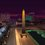# A Beautiful Problem

For some $m\in\mathbb N$, there are $2^m$ positive real numbers written on a board product of which is $1$. At each step, we can choose two numbers $a,b$ and replace both by $a+b$. Prove that after $2^{m-1}m$ steps the sum of all numbers on board will be at least $4^m$.Note by Jubayer Nirjhor
6 years, 2 months ago

This discussion board is a place to discuss our Daily Challenges and the math and science related to those challenges. Explanations are more than just a solution — they should explain the steps and thinking strategies that you used to obtain the solution. Comments should further the discussion of math and science.

When posting on Brilliant:

• Use the emojis to react to an explanation, whether you're congratulating a job well done , or just really confused .
• Ask specific questions about the challenge or the steps in somebody's explanation. Well-posed questions can add a lot to the discussion, but posting "I don't understand!" doesn't help anyone.
• Try to contribute something new to the discussion, whether it is an extension, generalization or other idea related to the challenge.

MarkdownAppears as
*italics* or _italics_ italics
**bold** or __bold__ bold
- bulleted- list
• bulleted
• list
1. numbered2. list
1. numbered
2. list
Note: you must add a full line of space before and after lists for them to show up correctly
paragraph 1paragraph 2

paragraph 1

paragraph 2

[example link](https://brilliant.org)example link
> This is a quote
This is a quote
    # I indented these lines
# 4 spaces, and now they show
# up as a code block.

print "hello world"
# I indented these lines
# 4 spaces, and now they show
# up as a code block.

print "hello world"
MathAppears as
Remember to wrap math in $$ ... $$ or $ ... $ to ensure proper formatting.
2 \times 3 $2 \times 3$
2^{34} $2^{34}$
a_{i-1} $a_{i-1}$
\frac{2}{3} $\frac{2}{3}$
\sqrt{2} $\sqrt{2}$
\sum_{i=1}^3 $\sum_{i=1}^3$
\sin \theta $\sin \theta$
\boxed{123} $\boxed{123}$

Sort by:

How does this work? I'm confused by the #WishfulThinking tag. Is the question correct?

The sum of all the numbers is invariant and equal to $2^m$ and can never be $4^m$

. Also, the number of numbers on the boards decreases by one every step. So after $2^m -1$ steps, there should only be $1$ number left on the board, after which no moves are possible. So you can't have $2^{m-1}*m$ moves since $2^{m-1}*m > 2^{m} -1$ for $m \geq 2$

- 6 years, 2 months ago

We replace "both" of $a,b$ by $a+b$ so $(a,b)$ changes to $(a+b,a+b)$. Thus the number of terms remains same.

- 6 years, 2 months ago

Ah. Okay. The question is still ambiguously worded though. A better wording would be " we replace each of $a,b$ with $(a+b)$"

Anyways, the problem seems easy. Just apply the "step" to the two largest numbers which has a sum of $\geq 2$. Each "step" doubles the sum of the numbers. Initial sum = $a+b$, final sum $= 2(a+b)$. Applying it $2^{m-1}m$ times, the sum of those two numbers is now $\geq 2^{2^{m-1}m}$. which is obviously greater than $2^{2m}$

- 6 years, 2 months ago

Not so fast! If initial terms are $a_1,...,a_{2^m}$ then initial sum is $a_1+\cdots+a_{2^m}$, while the sum after applying the step is $2a_1+2a_2+a_3+\cdots+a_{2^m}$. So the sum is not doubled.

- 6 years, 2 months ago

Sorry. I wasn't clear. I meant the sum of the largest two numbers when I said the sum will be doubled.

My solution was to just ignore the other numbers. Take only the two largest numbers. Their sum has to $\geq 2$. Now, one step doubles the sum of these two numbers. Applying the step $2^{m-1}*m$ times we see that the sum of these two numbers is now $\geq 2^{2^{m-1}m}$. The sum of the numbers we ignored is $\geq 0$. so doesn't matter. Clearly, the sum of all the numbers is now $2^{2^{m-1}m}$ which is clearly greater than $2^{2m}$.

- 6 years, 2 months ago

You don't decide which numbers to choose. The statement holds for any order.

- 6 years, 2 months ago

Any order? No it doesn't. Take the case where $m = 2$. Take $x_1, x_2, 2-x_1,2-x_2$. Sum of these $2^2$ numbers is $2^2$

Apply the step 4 times on $x_1, x_2$. Then the four numbers are $4(x_1+x_2),4(x_1+x_2), 2-x_1, 2-x_2$.

Sum of these numbers is $4 + 7(x_1+x_2)$. If we take $x_1,x_2$ arbitrarily small, it will not be greater than $16$.

- 6 years, 2 months ago

Sorry. A change has been made. The initial product of the numbers is $1$. No restriction on the sum.

- 6 years, 2 months ago